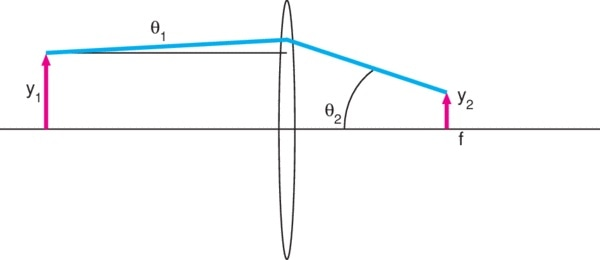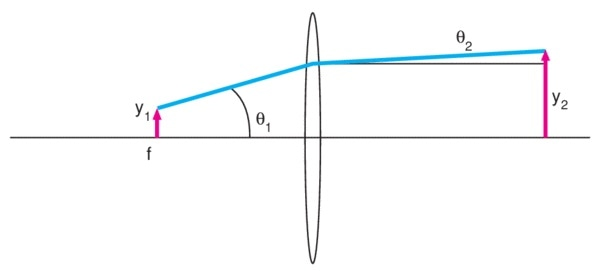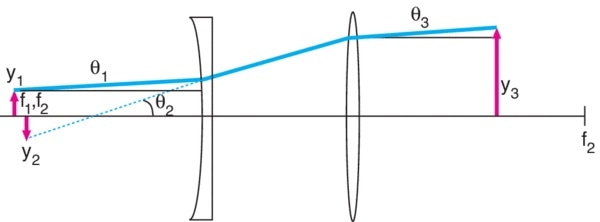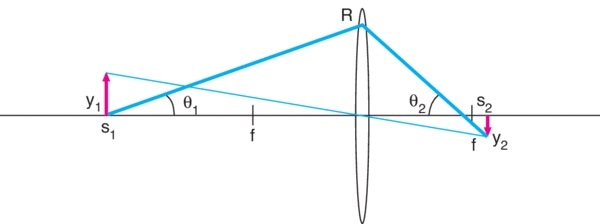# Focusing a Collimated Laser Beam

## Application 1: Focusing a Collimated Laser Beam

The first example we will look at is one typical to optics labs: focusing a laser beam onto a small spot. This is visualized in Figure 5, that shows a laser beam with radius y1 and divergence θ1 being focused by a lens of focal length f. we can see from the figure that θ2 = y1/f. From the optical invariant rule, it then follows that y2 = θ1f, as the product of radius and divergence angle must be constant.Figure 5

As a numerical example, we can examine the case of the output from a Newport R-31005 HeNe laser focused to a spot using a KPX043 Plano-Convex Lens. This HeNe laser has a beam diameter of 0.63 mm and a divergence of 1.3 mrad.

It should be considered that these are beam diameter and full divergence, so in the notation of our figure, y1 = 0.315 mm and θ1 = 0.65 mrad. The KPX043 lens has a focal length of 25.4 mm. Thus, at the focused spot, we have a radius θ1f = 16.5 µm. So, the diameter of the spot will be 33 µm.

This is a fundamental limitation on the minimum size of the focused spot in this application. As we have already assumed a flawless, aberration-free lens, any further improvement of the lens would not bring about an improvement in the spot size. The only route possible would be to use a shorter focal length lens or expand the beam.

If this can’t be done due to a limitation in the geometry of the optical system, then this is the minimum possible spot size. On top of this, diffraction may increase the minimum spot size (see Gaussian Beam Optics), but for this example we can ignore wave optics and only consider ray optics.

## Application 2: Collimating Light from a Point Source

Figure 6 shows another common application: the collimation of light from a very small source. This issue is often talked about in terms of collimating the output from a “point source.” This is difficult as there is no real-world example of a true point and the size of the source must be included in any calculation. The point source shown in figure 6 has a radius of y1 and has a maximum ray of angle θ1.

If the output from this source is collimated using a lens with focal length f, then the resulting beam will have radius y2 = θ1f and divergence angle θ2 = y1/f. It should be noted that whatever lens is used, the beam radius and beam divergence have a reciprocal relation. For example, to improve the collimation by a factor of two, the beam diameter should be increased by a factor of two.Figure 6

One of the typical applications for this is the collimation of the output from an Optical Fiber, so that will be used as the numerical example. The Newport F-MBB fiber has a core diameter of 200 µm and a numerical aperture (NA) of 0.37. The radius y1 of our source is then 100 µm. NA is defined in terms of the half-angle accepted by the fiber, so θ1 = 0.37.

If we again use the KPX043 , 25.4 mm focal length lens to collimate the output, the resulting beam will have a radius of 9.4 mm and a half-angle divergence of 4 mrad. This particular relation between the size and divergence of the beam is constant. If we wanted to reduce the beam diameter, we must accept that it will have a larger divergence. If we want the beam to remain collimated over a large distance, then we must in turn realize that this requires a larger beam diameter.

## Application 3: Expanding a Laser Beam

There are many applications in which the laser beam requires expansion, a task for which at least two lenses are needed. In Figure 7, a laser beam of radius y1 and divergence θ1 is expanded by a negative lens with focal length −f1. We know from Applications 1 and 2 that θ2 = y1/|−f1|, and the optical invariant tells us that the radius of the virtual image formed by this lens is y2 = θ1|−f1|.

This image is at the focal point of the lens, s2 = −f1, because a well-collimated laser yields s1 ~ ∞, so from the Gaussian lens equation s2 = f. By using an additional lens with a positive focal length f2 and keeping the two lenses apart by a distance equal to the sum of the two focal lengths −f1 +f2, results in a beam with a radius y3 = θ2f2 and divergence angle θ3 = y2/f2.Figure 7

The expansion ratio

y3/y1 = θ2f22|−f1| = f2/| −f1|

or the ratio of the focal lengths of the lenses. The expanded beam diameter

2y3 = 2θ2f2 = 2y1f2/|−f1|

The divergence angle of the resulting expanded beam

θ3 = y2/f2 = θ1|−f1|/f2

is reduced from the original divergence by a factor that is equal to the ratio of the focal lengths |-f1|/f2. So, to increase the beam diameter by a factor of five we would select two lenses whose focal lengths differ by a factor of five, and the divergence angle of the expanded beam would be 1/5th the original divergence angle.

To put this into context, consider a Newport R-31005 HeNe Laser with beam diameter 0.63 mm and a divergence of 1.3 mrad. Note that these are beam diameter and full divergence, so in the notation of our figure, y1 = 0.315 mm and θ1 = 0.65 mrad.

In order  to expand this beam by a factor of ten while reducing the divergence by a factor of ten, we require a plano-concave lens KPC043 with f1 = -25 mm and a plano-convex lens KPX109 with f2= 250 mm.

Since there is some variation between real lenses differ and thin lenses, the lens spacing should actually be the sum of the back focal lengths BFL1 + BFL2 = -26.64 mm + 247.61 mm = 220.97 mm. The expanded beam diameter

2y3 = 2y1f2/|-f1| = 2(0.315 mm)(250 mm)/|-25 mm|= 6.3 mm.

The divergence angle

To minimize the effect of aberrations, the ideal setup uses a plano-concave lens for the negative lens and a plano-convex lens for the positive lens with the plano surfaces facing each other. By only illuminating the central portion of the lens, we can further reduce aberrations, so choosing oversized lenses is often a good idea. This configuration is known as a Galilean beam expander. Another type of setup is known as Keplerian: this style uses two positive lenses, but results in a longer configuration than the Galilean design.

## Application 4: Focusing an Extended Source to a Small Spot

We will approach this application as an imaging problem rather than the focusing and collimation problems of the previous applications. Examples may include a situation where a fluorescing sample must be imaged with a CCD camera. Figure 8 shows the geometry of the application.

An extended source with a radius of y1 is located at a distance s1 from a lens of focal length f. From the figure we can see a ray incident upon the lens at a radius of R. We can take this radius R to be the maximal allowed ray, or clear aperture, of the lens.Figure 8

If s1 is large, then s2 will be close to f, from our Gaussian lens equation, so for the purposes of approximation we can take θ2 ~ R/f. Then from the optical invariant, we have

y2 = y1θ12 = y1(R/s1)(f/R) or y2 = 2y1(R/s1)f/#

where f/2R = f/D is the f-number, f/#, of the lens. For the image size to be made smaller, we could make f/# smaller, but we are limited to f/# = 1 or so. From there we can either decrease R (smaller lens or aperture stop in front of the lens) or increase s1.

However, each of those will lead to a restriction of the light gathered by the lens. If we either decrease R by a factor of two or increase s1 by a factor of two, it would decrease the total light focused at s2 by a factor of four due to the restriction of the solid angle subtended by the lens.This information has been sourced, reviewed and adapted from materials provided by Oriel Instruments.

## Citations

• APA

Oriel Instruments. (2018, September 10). Focusing a Collimated Laser Beam. AZoOptics. Retrieved on October 25, 2020 from https://www.azooptics.com/Article.aspx?ArticleID=1430.

• MLA

Oriel Instruments. "Focusing a Collimated Laser Beam". AZoOptics. 25 October 2020. <https://www.azooptics.com/Article.aspx?ArticleID=1430>.

• Chicago

Oriel Instruments. "Focusing a Collimated Laser Beam". AZoOptics. https://www.azooptics.com/Article.aspx?ArticleID=1430. (accessed October 25, 2020).

• Harvard

Oriel Instruments. 2018. Focusing a Collimated Laser Beam. AZoOptics, viewed 25 October 2020, https://www.azooptics.com/Article.aspx?ArticleID=1430.